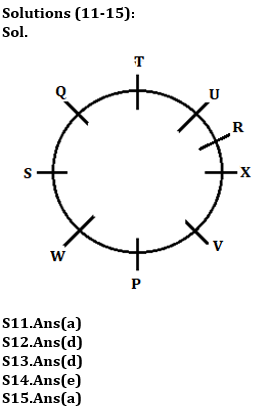Latest Banking jobs   »

# Reasoning Quiz For RBI Grade B Phase 1 2023-09th May

Directions (1-5): Following questions are based on the five words given below, Study the following words and answer the following question:

SOON CARS RAIN MINE NOON

Q1. If all the letters are arranged in alphabetical order within each of the words, then how many words will start with a vowel?
(a) One
(b) Three
(c) Two
(d) Four
(e) Five

Q2. If the given words are arranged in the order as they appear in a dictionary from left to right, then which of the following word will be second from the right end?
(a) RAIN
(b) MINE
(c) SOON
(d) NOON
(e) CARS

Q3. How many letters are in between the first letter of the first word from the left end and the second letter of the third word from the right end (According to the English alphabet)?
(a) 12
(b) 17
(c) 15
(d) 19
(e) 18

Q4. If in each of the given words, every consonant is changed to its previous letter and every vowel is changed to its next letter according to the English alphabetical series, then in how many words, thus formed, at least one vowel will appear?
(a) One
(b) Two
(c) Three
(d) More than three
(e) None

Q5. If the given words are arranged in the order as they appear in a dictionary from right to left, which of the following word will be second from the right end?
(a) RAIN
(b) MINE
(c) NOON
(d) SOON
(e) CARS

Directions (6-10): These questions are based on the following five numbers.

234 436 517 625 397

Q6. If all the digits of numbers are arranged in ascending order within each of the number, then which of the following is third lowest number thus formed?
(a) 234
(b) 517
(c) 397
(d) 625
(e) None of these

Q7. If 1st digit of highest number is divided by 2nd digit of 2nd highest number, then what will be the resultant?
(a) 2
(b) 3
(c) 4
(d) 9
(e) None of these

Q8. If 1st digit is replaced with 2nd digit, 2nd digit is replaced with the 3rd digit and 3rd digit is replaced with the 1st digit within the number, then which number thus formed will be the lowest among all?
(a) 517
(b) 625
(c) 436
(d) 234
(e) None of these

Q9. What is the sum of 3rd digit of 2nd highest number and 1st digit of lowest number?
(a) 7
(b) 6
(c) 4
(d) 9
(e) None of these

Q10. If 1st digit of 2nd highest number is multiplied by 1st digit of lowest number, then what will be the resultant?
(a) 15
(b) 16
(c) 10
(d) 14
(e) None of these

Directions (11-15): Study the information carefully and answer the questions given below.
Nine persons P, Q, R, S, T, U, V, W and X are sitting in a circle but not necessary in same order. All of them face inside.
R sits third to the right of P. Two persons sit between R and Q. U sits second to the right of X who is not immediate neighbor of P. Two persons sit between T and W. S is third to the left of V. S is not immediate neighbor of T and P who does not sit immediate right of V.

Q11. How many persons sit between S and P when counted from left of P?
(a) One
(b) Two
(c) Four
(d) Three
(e) None

Q12. Who among the following sits 4th to right of W?
(a) V
(b) P
(c) S
(d) R
(e) None of these

Q13. How many persons sit between Q and X when counted from right of Q?
(a) Two
(b) One
(c) Three
(d) More than three
(e) None

Q14. Who sits second to the right of U?
(a) W
(b) T
(c) V
(d) P
(e) None of these

Q15. If W and T interchange their positions, then who sits second to the right of W?
(a) S
(b) P
(c) R
(d) T
(e) None of these

Solutions

Solutions (1-5):
S1. Ans. (b)
Sol. SOON CARS RAIN MINE NOON
NOOS ACRS AINR EIMN NNOO

S2. Ans. (a)
Sol. Arrangement according to dictionary order from left to right,
CARS MINE NOON RAIN SOON

S3. Ans. (b)
Sol. Number of letters between S and A is 17.

S4. Ans. (e)
Sol. SOON CARS RAIN MINE NOON
RPPM BBQR QBJM LJMF MPPM

S5. Ans. (b)
Sol. Arrangement according to dictionary from right to left,
SOON RAIN NOON MINE CARS

Solutions (6-10):
S6. Ans.(d)
Sol. 234 436 517 625 397
234 346 157 256 379

S7. Ans.(e)
Sol. If 1st digit of highest number is divided by 2nd digit of 2nd highest number,
then the resultant is=6/1=6

S8. Ans.(a)
Sol. 234 436 517 625 397
342 364 175 256 973

S9. Ans.(d)
Sol. Addition of 3rd digit of 2nd highest number and 1st digit of lowest number=7+2=9

S10. Ans.(c)
Sol. If 1st digit of 2nd highest number is multiplied by 1st digit of lowest number, then the resultant is= 5*2=10## FAQs

### What is the selection process of the Bank Clerk?

There are 4 sections in the RBI Grade B Phase 1 Exam i.e. English Language, General Awareness, Quantitative Aptitude & Reasoning.

#### Congratulations!Union Budget 2023-24: Free PDF